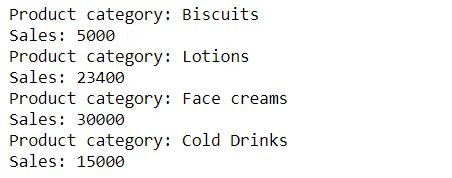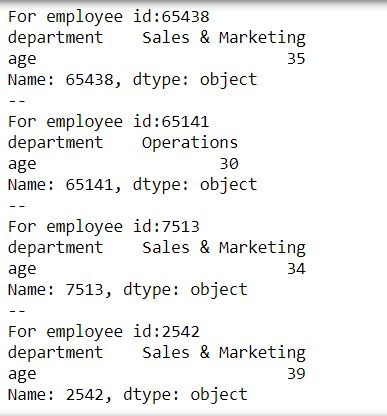Search
•Ekta Aggarwal

# For loops for different iterables.

In this tutorial we shall try to understand how for loop is modified for various iterables (like lists, tuples, dictionaries, zipped objects etc.).

Topics covered:

General syntax of a for loop:

```for iterator in iterable:
<Do Something>```

In Python indentation means a lot. Thus you need to have same number of spaces for a code written in for loops

### For loop with Strings:

When for loop is iterated over a string then it returns the letters of the string with each iteration

In the following code: Our iterable is our string 'salary'.

For loop firstly takes i = 's' and then prints it, then it takes i = 'a' and prints it. This process goes on till all of the letters in the string get exhausted.

```for i in 'salary':
print(i)```### For loop with lists:

To understand how for loop behaves with a list, let us create our list named salary:

`salary = [1000,2000,3000,4000]`

In the following code our iterator i firstly takes first element of our list i.e. i = 1000 and prints it,

then i takes the value 2000 and prints it. This is done till i reaches the last element of our list

```for i in salary:
print(i)```### enumerate

Sometimes we need to keep track of the number of iterations. In case of lists this can be done so using enumerate( ) function

```salary = [1000,2000,3000,4000]
list(enumerate(salary))```

Enumerate function zips the iteration number with each element in a list to form a tuple.We can iterate over a list using its index by defining 2 iterators in for loop and our iterable would be enumerate(list_name)

In the following code our 2 iterators are: index, value

We are iterating upon : enumerate(salary)

```for index, value in enumerate(salary):
print(index)
print(value)
print('--')```enumerate( ) provides the option to set the starting value of the index. By default start = 0.

In the following code we have defined that our indexing should start from 1.

```for index, value in enumerate(salary,start = 1):
print(index)
print(value)
print('--')```### Iterating over zipped objects

Let us firstly create our 2 lists which we will zip together

```product_category = ['Biscuits','Lotions','Face creams','Cold Drinks']
sales = [5000,23400,30000,15000]```

We can iterate over multiple iterables in one single for loop using zipped objects (avoiding multiple for loops)

In the following code we have created two iterator objects pc and s which iterate over various values of zipped object one by one.

```for pc,s in zip(product_category,sales):
print('Product category: ' + str(pc))
print('Sales: ' + str(s))```### Iterating over data

Sometimes loading heavy files can be too much time consuming thus we can load the data in chunks.

Let us consider this csv file:

HR_data
.csv

`import pandas as pd`

Suppose we just want to get the total of age of all the employees and do not wish to retain the data later on thus we can iterate over our csv file by defining chunksize = 1000 in read_csv function - which tells Python that data should be read in chunks of 1000 rows and then for each chunk sum of age should be appended to our empty list result.

```result = []
for chunk in pd.read_csv("HR_data.csv",chunksize = 1000):
result.append(sum(chunk['age']))

sum(result)```### Iterating over dictionaries

Let us create our dictionary:

```my_dict = {'U.S.A' : 'Washington D.C',
'U.K.' : 'London',
'Japan':'Tokyo',
'Russia':'Moscow'}```

Our dictionary has items - which are the combination of key - value pairs:

`my_dict.items()`In dict_name.items( ) the first element is the key name and second element is the value name

To iterate over keys and values in our dictionary we define our for loop as:

Iterator variable names: key, value

Iterator over my_dict.items( )

```for key,value in my_dict.items():
print(key)
print(value)
print('--')```We can use other iterator variable names: country and capital. The output would be same as above.

```for country,capital in my_dict.items():
print(country)
print(capital)
print('--')```

### iterrows

Let us firstly read and save our csv file and define index_col = 0, telling Python that 1st column is the row names.

```hrdata = pd.read_csv("HR_data.csv",index_col = 0)

Let us firstly see what happens if we iterate over hrdata?

```for i in hrdata:
print(i)```

It returns the column names:To iterate over various rows and column entries in a data frame we use iterrows function

Here we have two iterator variables: row and col

and our iterable object is hrdata.iterrows( )

```for row,col in hrdata.iterrows():
print("For employee id:" + str(row))
print(col)
print('--')```

Here row is taking the row name (our employee id)

while col is denoting the values for each row-col combinationTask: Fetch the row and column entries only for department column using iterrows

To achieve this we have filtered our iterator col by 'department'

```for row,col in hrdata.iterrows():
print("For employee id:" + str(row))
print(col['department'])
print('--')```Task: Fetch the row and column entries only for department and age columns using iterrows

To achieve this we have filtered our iterator col by providing a list ['department','age']

```for row,col in hrdata.iterrows():
print("For employee id:" + str(row))
print(col[['department','age']])
print('--')```See All